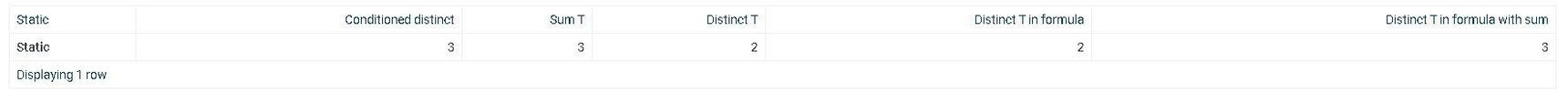0

# Create a calculated formula based on distinct values

We are trying to create a logic where we want the distinct count of a dimension when a measure equals a certain value

problem:
*when a distinct function is enclosed into a sum function it works as normal count
*when creating a formula column with if statement, it must be enclosed into a sum function to work, and we face the problem that the distinct works as normal count

below is a sample table to demonstrate:
create table Test_Table (static nvarchar(10),t nvarchar(10),n int)

insert into Test_Table values('Static','a',1)
insert into Test_Table values('Static','a',1)
insert into Test_Table values('Static','a',0)
insert into Test_Table values('Static','b',1)
insert into Test_Table values('Static','b',0)

formula:

sum(
if(
schema.Test_Table.n = 1,
distinct(
ValU.Test_Table.t
),

)• Oldest first